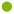Special Properties of DecimalsDensity of the real numbersWhy left-to-right comparison works Uniqueness of decimal expansions.### Density of the real numbers

The first number concepts that children learn are discrete. The counting numbers 1,2,3, 4, . . . describe collections of objects, not measures of continuous quantities such as length, weight or time.

Unlike the set of counting numbers, both the sets of decimals and fractions have the property that between any two there is another one. In between the two whole numbers 2 and 3, for example, there is no whole number. But in between any two fractions there is always another fraction and in between two decimals there is always another decimal.

Some students do not realise that the decimals and fractions have this property. Students who think of decimals in terms of money, for example, often do not realise that there are distinct decimals with more than two decimal places. Student who exhibit this money thinking think that decimals such as 0.45 and 0.4502 are exactly the same. They cannot tell which is bigger. They see the extra decimal places as only some sort of "error", perhaps generated in a calculator. They think there are really only 100 decimal numbers between any two whole numbers, rather than an infinite number. Their picture of the number line is as a series of isolated points, close together, not as a continuous line without any gaps.

Note: More information about the properties of the set of all fractions (rational numbers) and the set of all decimals (real numbers) and the differences between them.### Why left-to-right comparison works

Children need to understand, on an intuitive level, why left-to-right comparison of decimal or whole numbers works. This is such a familiar idea that it is easy to overlook it and forget to ensure that children understand why this is so, at some level.

Whereas there are many equivalent fractions, there is really only one way of writing a given number as a whole number or decimal. The fraction 1/2 can also be written as 2/4 or 3/6 or 4/8 or 147/254 or 123456/246912 which look quite different but the decimal 0.5 can only be written as 0.5 or 0.50 or 0.500 or 0.50000000000 etc. It always looks nearly the same. (There is one exception with infinite strings of repeating nines - more information).

This means that if two decimals differ in any column (apart from final zeros) then they are definitely different in value. It also means that, no matter what numbers are present to the right of a column, they can never add up to change the amount in that column.

The importance of this idea is that with both whole numbers and decimals, you use the left-to-right digit-by-digit comparison strategy to see which of two decimals is the larger. The mathematical ideas are fully explained here.